Courses

# Problems for Practice - Complex Numbers Mathematics Notes | EduRev

## Algebra for IIT JAM Mathematics

Created by: Veda Institute

## Mathematics : Problems for Practice - Complex Numbers Mathematics Notes | EduRev

The document Problems for Practice - Complex Numbers Mathematics Notes | EduRev is a part of the Mathematics Course Algebra for IIT JAM Mathematics.
All you need of Mathematics at this link: Mathematics

Complex Numbers - Practice Problems
Q.1. (4−5i)(12+11i)(4−5i)(12+11i)
Ans. We know how to multiply two polynomials and so we also know how to multiply two complex numbers. All we need to do is “foil” the two complex numbers to get,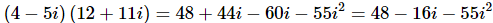All we need to do to finish the problem is to recall that i2=−1. Upon using this fact we can finish the problem.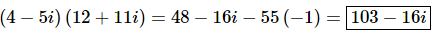Q.2. (−3−i)−(6−7i)
Ans. We know how to subtract two polynomials and so we also know how to subtract two complex numbers.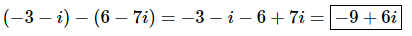Q.3. (1+4i)−(−16+9i)
Ans.
We know how to subtract two polynomials and so we also know how to subtract two complex numbers.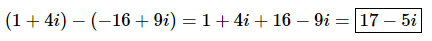Q.4. 8i(10+2i)
Ans. We know how to multiply two polynomials and so we also know how to multiply two complex numbers. All we need to do is distribute the 8i to get,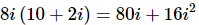All we need to do to finish the problem is to recall that i2=−1. Upon using this fact we can finish the problem.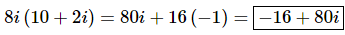Q.5. (−3−9i)(1+10i)
Ans. We know how to multiply two polynomials and so we also know how to multiply two complex numbers. All we need to do is “foil” the two complex numbers to get,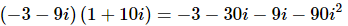All we need to do to finish the problem is to recall that i2=−1. Upon using this fact we can finish the problem.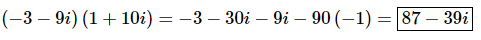Q.6. (2+7i)(8+3i)
Ans. We know how to multiply two polynomials and so we also know how to multiply two complex numbers. All we need to do is “foil” the two complex numbers to get,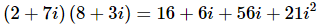All we need to do to finish the problem is to recall that i2=−1. Upon using this fact we can finish the problem.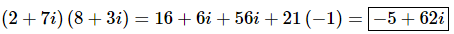Q.7.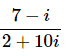Ans. Because standard form does not allow for i’s to be in the denominator we’ll need to multiply the numerator and denominator by the conjugate of the denominator, which is 2−10i.
Multiplying by the conjugate gives,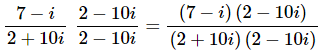Now all we need to do is do the multiplication in the numerator and denominator and put the result in standard form.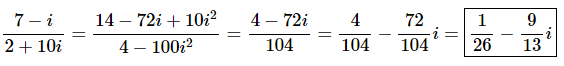Q.8.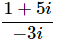Ans. Because standard form does not allow for ii’s to be in the denominator we’ll need to multiply the numerator and denominator by the conjugate of the denominator, which is 3i.
Multiplying by the conjugate gives,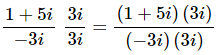Now all we need to do is do the multiplication in the numerator and denominator and put the result in standard form.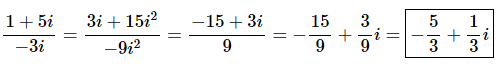Q.9.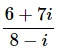Ans. Because standard form does not allow for i’s to be in the denominator we’ll need to multiply the numerator and denominator by the conjugate of the denominator, which is 8+i.
Multiplying by the conjugate gives,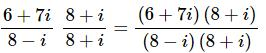Now all we need to do is do the multiplication in the numerator and denominator and put the result in standard form.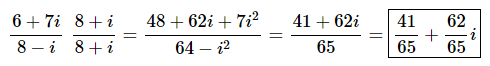Offer running on EduRev: Apply code STAYHOME200 to get INR 200 off on our premium plan EduRev Infinity!

58 docs

,

,

,

,

,

,

,

,

,

,

,

,

,

,

,

,

,

,

,

,

,

;